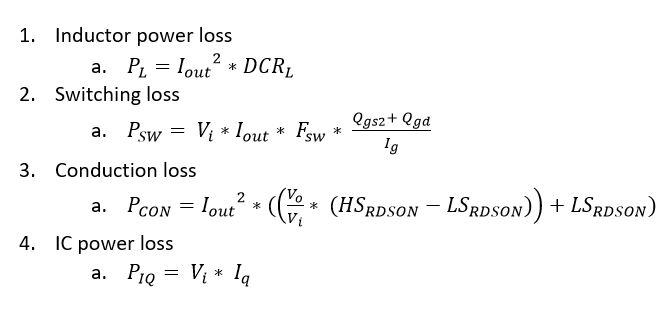# MP1654 power dissipation formula

I would like to know MP1654 power dissipation formula to calculate power loss.

can you share with me ?

Hi Ray,

To estimate power loss it’s good to break it down into its main components. In decreasing order of significance (typically):We simply add up all these losses to find the total power dissipation, which we can use to estimate overall efficiency.

You won’t find the Qgs2/Qgd/Ig terms in any datasheets though. This can be roughly approximated by using the efficiency plots in the datasheets and accounting for all sources of power dissipation to back-solve for this final term. It’s only an approximation, but it works well enough.

1 Like